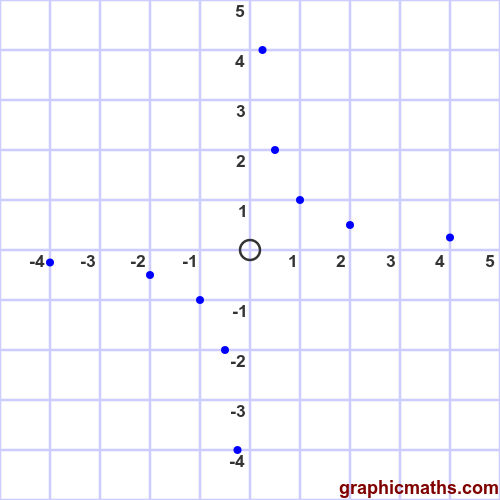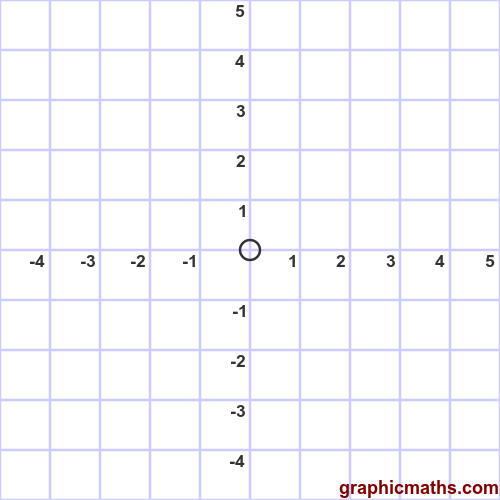# Rectangular hyperbola example

Martin McBride, 2020-09-12
Tags parametric equation hyperbola
Categories coordinate systems pure mathematics

A rectangular hyperbola has the parametric equations:

\begin{align} x = c t\newline y = \frac{c}{t} \end{align}

Where $c$ is a positive constant, and $t$ is the independent variable.

We can plot this curve by calculating the values of $x$ and $y$ for various values of $t$, and drawing a smooth curve through them.

## Curve for c = 1

Assuming $a = 1$, the parametric equations simplify to:

\begin{align} x = t\newline y = \frac{1}{t} \end{align}

The values are shown in the following table, for $t$ in the range -3 to +3:

t x y
-4 -3 -0.25
-2 -2 -0.5
-1 -2 -1
-0.5 -0.5 -2
0 0 undefined
0.5 0.5 2
1 1 1
2 2 0.5
4 4 0.25

Here are the points plotted on a graph:This curve is actually a standard reciprocal curve, as shown here.

The curve can be drawn by plotting the points and drawing a smooth line through them. Notice that the curve value is undefined for $t = 0$: